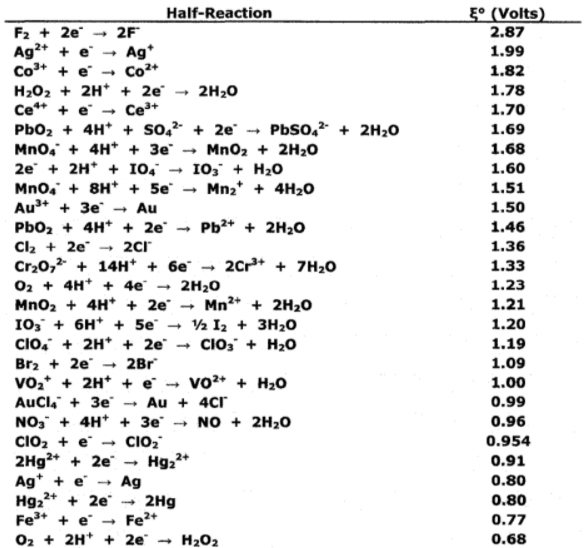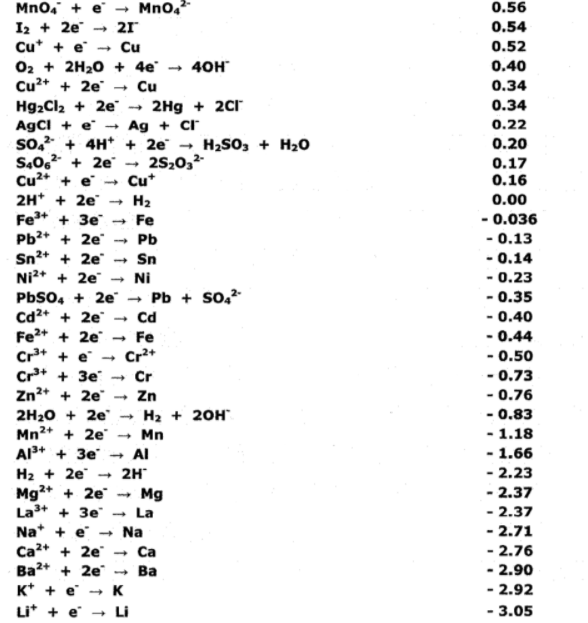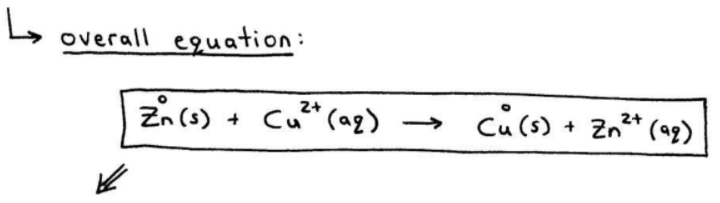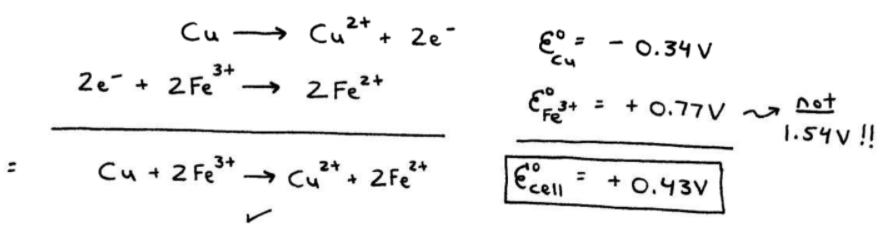# S17E2 - Cell Potential Calculations and Line Notation

## How to Calculate Galvanic Cell Potentials (Ecell)

We must first consider the standard reduction potentials for each half-reaction:

➞  E°cell

➞  values are listed in Table 17-1 of my *Chemistry Video Notes,* and also below...

### Table 17-1 = Standard Reduction Potentials (in Volts) at 25°C (298K) for Many Common Half-Reactions.-----

ex:  Why is the voltage 1.10 V in the redox reaction occurring in the galvanic cell below?The overall balanced redox equation is:

Zn(s)   +   Cu2+(aq)   →   Cu(s)   +   Zn2+(aq)Anode - where oxidation occurs:

Zn   →   Zn2+   +   2e-   ;   E°Zn = + 0.76 V  (from Table 17-1)

Cathode - where reduction occurs:

Cu2+   +   2e-   →   Cu   ;   E°Cu2+ = + 0.34 V  (from Table 17-1)

---

So, overall we have:

E°cell  =  E°Zn  +  E°Cu2+NOTE:  If the half-reaction is reversed in Table 17-1, you must change the sign (+/-) of E°, but if the half-reaction is multiplied through by an integer, the value of E° doesn't change!!

=====

## Cell Potential Calculations (Ecell)

ex:  Calculate the overall cell potential of the following reaction:

Cu(s)   +   2Fe3+(aq)   →   Cu2+(aq)   +   2Fe2+(aq)

➞ break up into half-reactions...=====

## Line Notation

When describing a galvanic cell (electrochemical cell), line notation is used.

➞ the anode compartment is listed on the LEFT.
➞ the cathode compartment is listed on the RIGHT.

ex:  A few sample problems back, we had an example with zinc and copper:

Zn(s)   +   Cu2+(aq)   →   Cu(s)   +   Zn2+(aq)

Here it is again, in an easy-to-follow handwritten format...=====

In my next post on SECTION 17 - Electrochemistry, my 3rd article on this subject,

Be sure to check it out!...